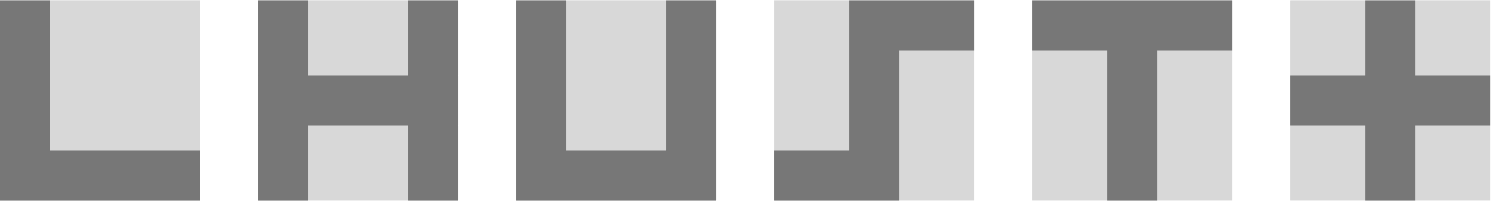# Problems of the Week

Contribute a problem

# 2018-11-12 Basic

When will it pop?

Six dark-grey symbols (L, H, U, S, T, $+$) are drawn on six identical pieces of square paper.

How many of these symbols have the same perimeter as the square paper itself?You are given two integers $J$ and $K.$

At most, how many of the following statements can be simultaneously true?

• $J + K$ is an odd number.
• $J - K$ is an odd number.
• $J \times K$ is an odd number.
• $J \div K$ is an odd number.

I am looking for a positive integer greater than 9 such that the product of all of its digits is equal to the integer itself:

$\underbrace{{\color{#D61F06}a} \times {\color{#3D99F6}b} \times {\color{#20A900}c} \times \cdots \times {\color{#302B94}x} \times {\color{#69047E}y} \times {\color{#E81990}z} = \overline{{\color{#D61F06}a}{\color{#3D99F6}b}{\color{#20A900}c} \ldots {\color{#302B94}x}{\color{#69047E}y}{\color{#E81990}z}}}_{\text{The product of the digits is equal to the integer itself.}}.$

Are there any positive integers that satisfy this condition?

$\sqrt1,\ \sqrt{11},\ \sqrt{111},\ \sqrt{1111},\ \sqrt{11111},\ \ldots$

Is it true that $\sqrt{1}$ is the only integer in this list of numbers?

×